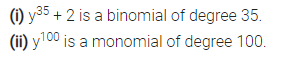# Give one example each of a binomial of degree 35, and of a monomial of degree 100

Give one example each of a binomial of degree 35, and of a monomial of degree 100.